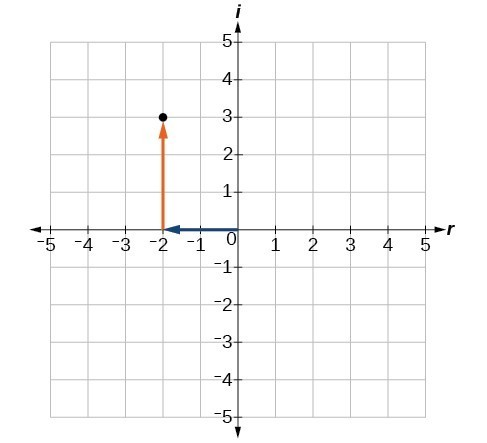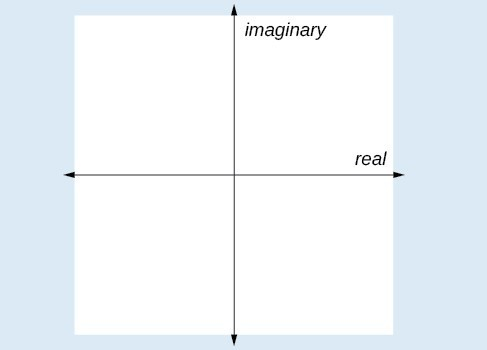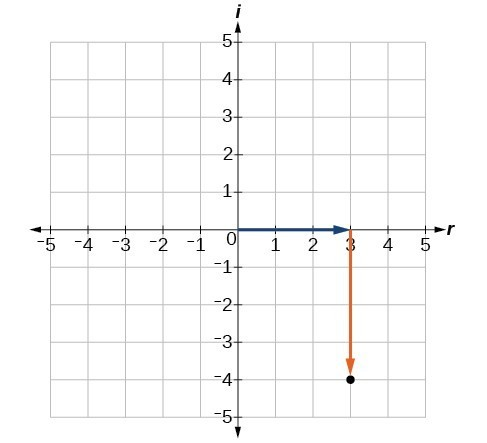## Plot complex numbers on the complex plane

We cannot plot complex numbers on a number line as we might real numbers. However, we can still represent them graphically. To represent a complex number we need to address the two components of the number. We use the complex plane, which is a coordinate system in which the horizontal axis represents the real component and the vertical axis represents the imaginary component. Complex numbers are the points on the plane, expressed as ordered pairs (a, b), where a represents the coordinate for the horizontal axis and b represents the coordinate for the vertical axis.Figure 2

Let’s consider the number $-2+3i\\$. The real part of the complex number is –2 and the imaginary part is 3i. We plot the ordered pair $\left(-2,3\right)\\$ to represent the complex number $-2+3i\\$.

### A General Note: Complex PlaneFigure 3

In the complex plane, the horizontal axis is the real axis, and the vertical axis is the imaginary axis.

### How To: Given a complex number, represent its components on the complex plane.

1. Determine the real part and the imaginary part of the complex number.
2. Move along the horizontal axis to show the real part of the number.
3. Move parallel to the vertical axis to show the imaginary part of the number.
4. Plot the point.

### Example 2: Plotting a Complex Number on the Complex Plane

Plot the complex number $3 - 4i\\$ on the complex plane.

### Solution

The real part of the complex number is 3, and the imaginary part is –4i. We plot the ordered pair $\left(3,-4\right)\\$.Figure 4

### Try It 2

Plot the complex number $-4-i\\$ on the complex plane.

Solution